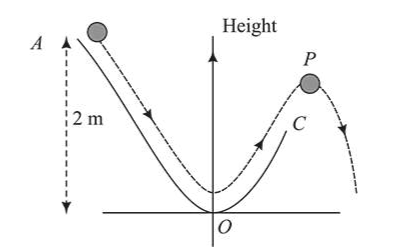# A particle (m=1 kg) slides down a frictionless track`
Question:

A particle $(m=1 \mathrm{~kg})$ slides down a frictionless track

(AOC) starting from rest at a point $A$ (height $2 \mathrm{~m}$ ).

After reaching $C$, the particle continues to move freely

in air as a projectile. When it reaching its highest

point $P$ (height $1 \mathrm{~m}$ ), the kinetic energy of the particle

(in $J$ ) is: $($ Figure drawn is schematic and not to scale;

take $g=10 \mathrm{~ms}^{-2}$ )Solution:

$(10.00)$

Kinetic energy $=$ change in potential energy of the

particle,

$\mathrm{KE}=\mathrm{mg} \Delta \mathrm{h}$

Given, $m=1 \mathrm{~kg}$

$\Delta h=\mathrm{h}_{2}-\mathrm{h}_{1}=2-1=1 m$

$\therefore K E=1 \times 10 \times 1=10 J$